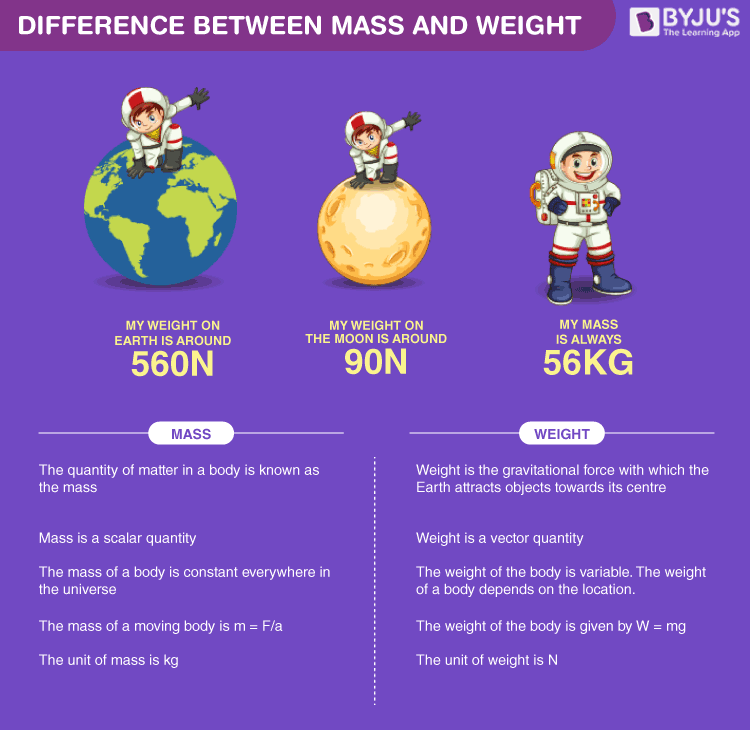# Difference Between Mass and WeightThe difference between mass and weight is one of the most frequently asked questions. Some students often use the terms mass and weight interchangeably, which is completely wrong. There are several differences between mass and weight that are discussed here in detail.

Before going to the mass and weight difference, it is important to know what they are and how they are related. To learn about mass and weight in detail, visit mass and weight. Here, the comparisons and differences between mass and weight with examples are given in the tabular form below.

## Difference Between Mass and Weight

 Sl. No. Differentiating Property Mass Weight 1 Definition Mass is simply the measure of the amount of matter in a body. Weight is the measure of the amount of force acting on a mass due to acceleration due to gravity. 2 Denotation Mass is denoted by “M”. Weight is denoted by “W”. 3 Formula Mass is always constant for a body and there are several formulas to calculate mass. One way to calculate mass is: Mass = volume × density Weight is the measure of the gravitational force acting on a body. Weight can be calculated from the following formula: Weight = mass × acceleration due to gravity 4 Quantity Type Mass is a base quantity. Mass only has magnitude and so, it is a scalar quantity. Weight is a derived quantity. Weight has both magnitude and direction (towards the centre of gravity) and so, it is a vector quantity. 5 Unit of Measurement The SI unit of mass is Kilogram (Kg). The SI unit of weight is Newton (N). 6 Gravitational Effect Mass does not depend upon gravity and is constant everywhere. Mass can never be zero. Weight is dependent on gravity and so, it varies from place to place. Weight can be zero where there is no gravity (like space). 7 Measuring Instrument Mass can be easily measured using any ordinary balance like beam balance, lever balance, pan balance, etc. Weight can be measured by a spring balance or by using its formula.

These were the main differences between mass and weight which many students are confused about. It is important to know about this topic in detail as it is a crucial part of the mechanics part in physics. Often questions based on the mass and weight differences are included in the competitive exams also.

Students are also required to know the basics of mass and weight thoroughly. Also, the formulas to determine weight and mass is crucial from the examination point of view. Check the articles given below to understand weight, mass and their formulas easily.

Important Articles:

See the video below to learn about the mystery of mass change.Stay tuned to BYJU’S to get more such Physics lessons in an easy and detailed way.

Test your knowledge on Mass and weight differences

1. Is weight of a body equal at all locations on Earth ? If not please let me know me where both mass and weight are same.

• No, weight is not equal at all locations on Earth. This is because weight is dependent on gravity and gravity varies. You can read about the causes for variations in gravity here.

2. Hii this is satyam and it was very helpful for me and help me in doing my homework thanks byjus for this requirement

3. thank you, the info is so helping and easy to understand.

5. It proved literally very helpful!

6. Very effective learning support, I appreciate it.

7. Very nice ❤️❤️❤️❤️❤️❤️❤️

8. Very easy to understand 🙂🙂

9. Esay to understand and very helpful !!❤

10. Very nice

11. When we buy something from market by weighing in a balance , what is actually measured and reasons therefore?

• When we buy things at the market and use a scale to measure them, we’re actually measuring their mass. If we use a balance, we compare the mass of the object to a known standard. It’s important to note that we’re not measuring the weight of the object because we’re not comparing the force of gravity acting on it.